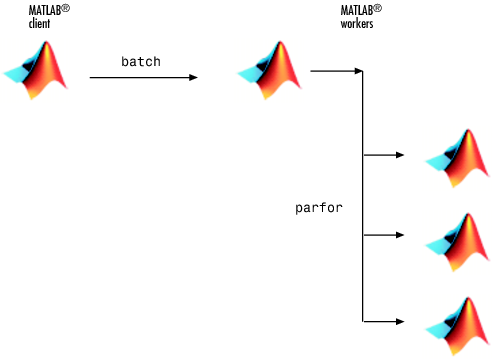Documentation

## Run Batch Parallel Jobs

### Run a Batch Job

To offload work from your MATLAB® session to run in the background in another session, you can use the `batch` command inside a script.

1. To create the script, type:

`edit mywave`
2. In the MATLAB Editor, create a `for`-loop:

```for i = 1:1024 A(i) = sin(i*2*pi/1024); end```
3. Save the file and close the Editor.

4. Use the `batch` command in the MATLAB Command Window to run your script on a separate MATLAB worker:

`job = batch('mywave')`5. The `batch` command does not block MATLAB, so you must wait for the job to finish before you can retrieve and view its results:

`wait(job)`
6. The `load` command transfers variables created on the worker to the client workspace, where you can view the results:

```load(job,'A') plot(A)```
7. When the job is complete, permanently delete its data and remove its reference from the workspace:

```delete(job) clear job```

`batch` runs your code on a local worker or a cluster worker, but does not require a parallel pool.

You can use `batch` to run either scripts or functions. For more details, see the `batch` reference page.

### Run a Batch Job with a Parallel Pool

You can combine the abilities to offload a job and run a loop in a parallel pool. This example combines the two to create a simple batch `parfor`-loop.

1. To create a script, type:

`edit mywave`
2. In the MATLAB Editor, create a `parfor`-loop:

```parfor i = 1:1024 A(i) = sin(i*2*pi/1024); end```
3. Save the file and close the Editor.

4. Run the script in MATLAB with the `batch` command. Indicate that the script should use a parallel pool for the loop:

`job = batch('mywave','Pool',3)`

This command specifies that three workers (in addition to the one running the batch script) are to evaluate the loop iterations. Therefore, this example uses a total of four local workers, including the one worker running the batch script. Altogether, there are five MATLAB sessions involved, as shown in the following diagram.5. To view the results:

```wait(job) load(job,'A') plot(A)```

The results look the same as before, however, there are two important differences in execution:

• The work of defining the `parfor`-loop and accumulating its results are offloaded to another MATLAB session by `batch`.

• The loop iterations are distributed from one MATLAB worker to another set of workers running simultaneously (`'Pool'` and `parfor`), so the loop might run faster than having only one worker execute it.

6. When the job is complete, permanently delete its data and remove its reference from the workspace:

```delete(job) clear job```

Watch now